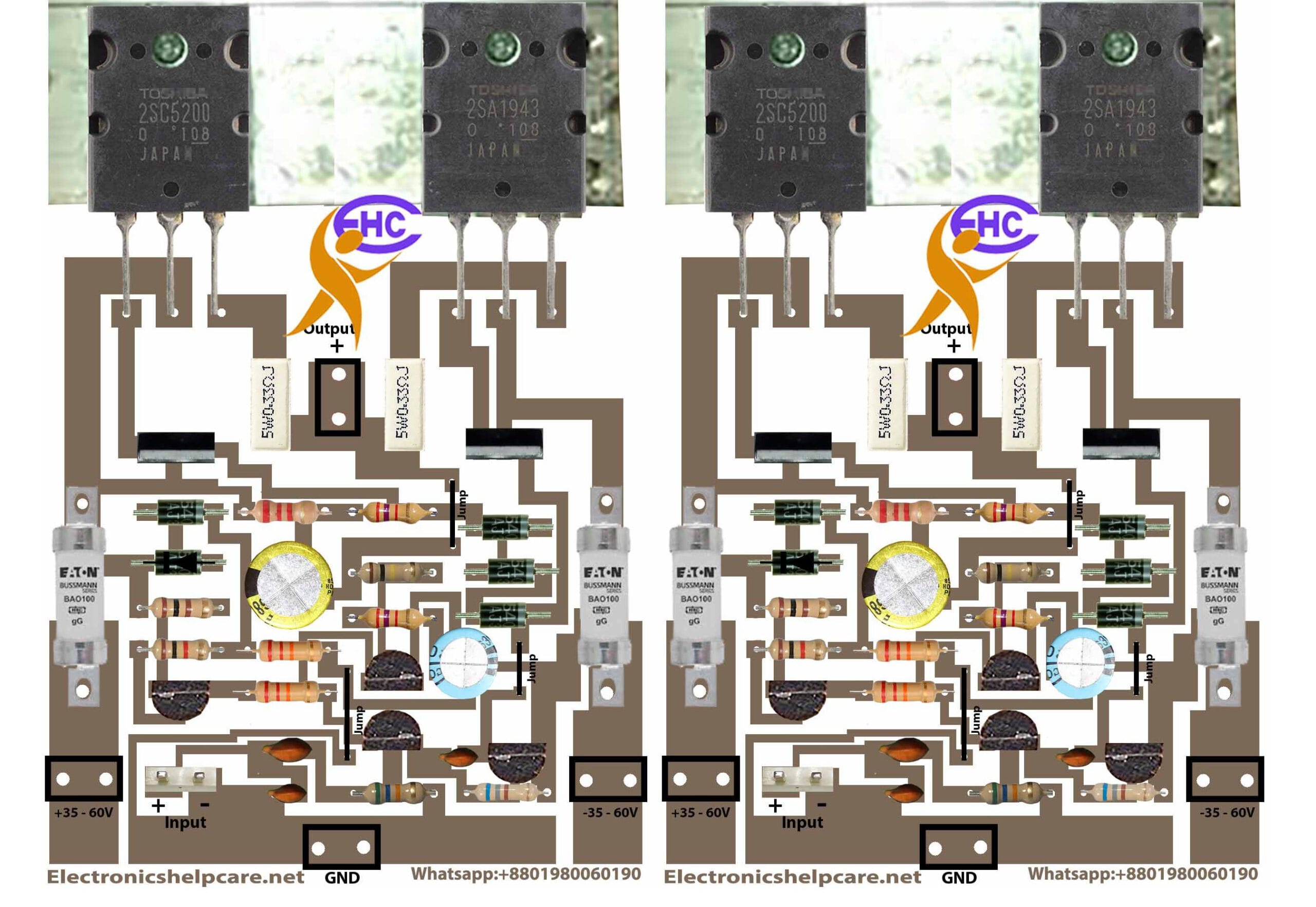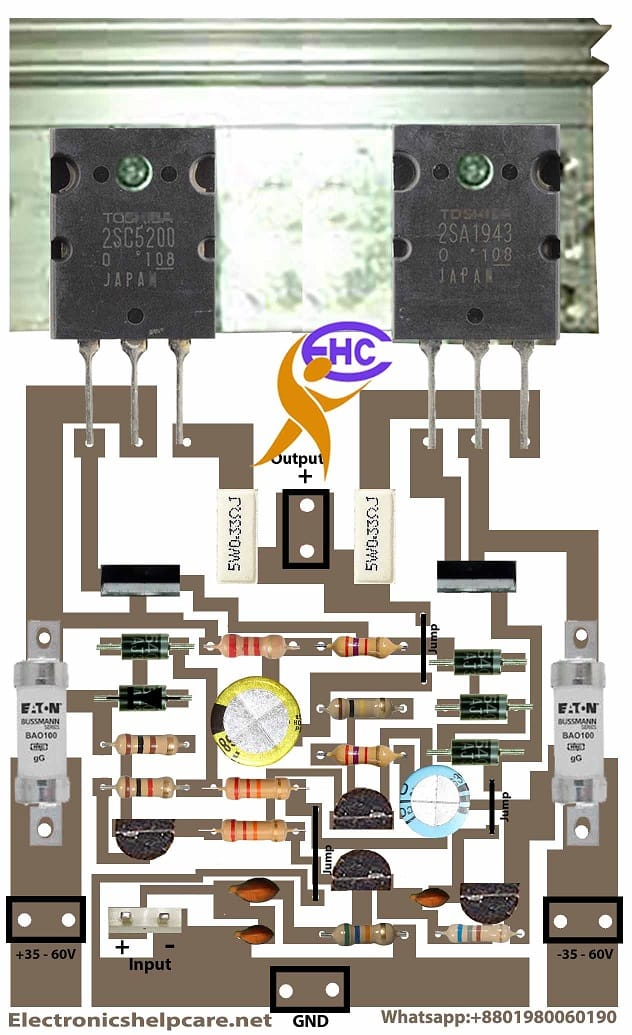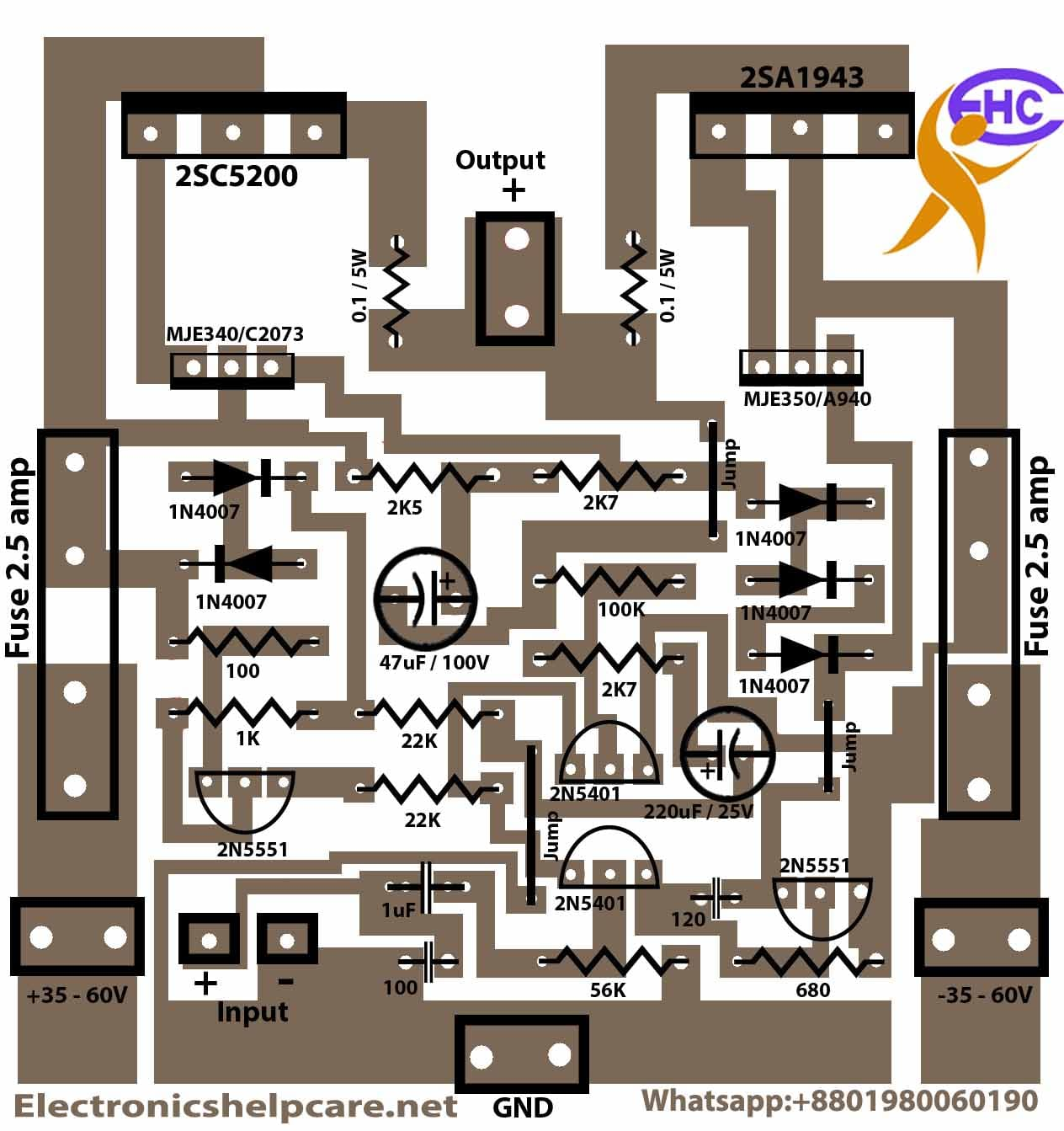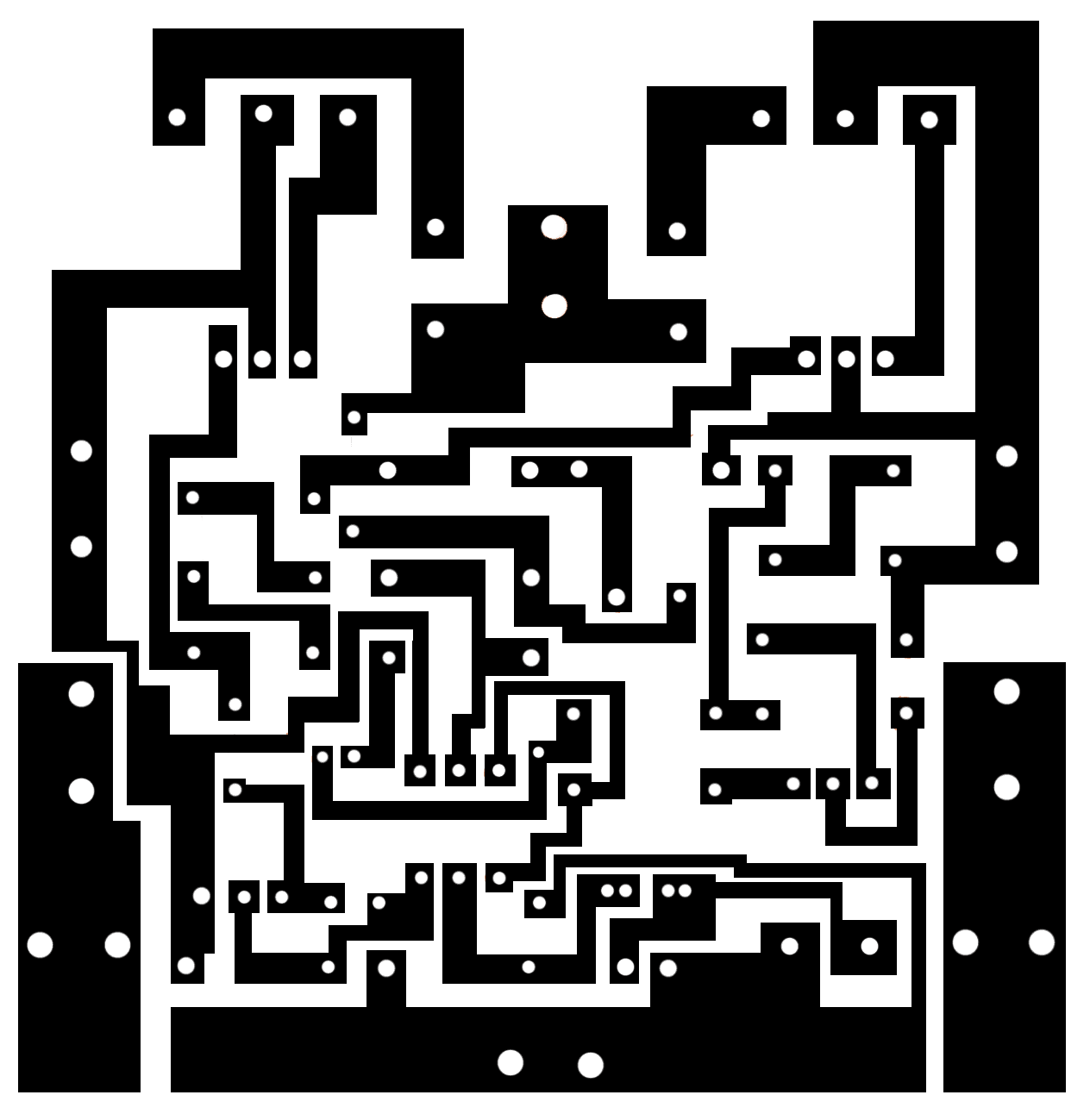# how to make amplifier board?

## how to make an amplifier board:

This is a 2sc5200 2sa1943 amplifier board with 200 Watts. this circuit is a mono amplifier circuit diagram. but we can use it in stereo mode by using two circuit boards. In this circuit, we can use 24-0-24 to 50-0-50 voltage in this circuit. and the voltage ampere is 4 . because one transistor can take 1.3 ampers. if we use 4 amperes then we can get 200 watts. voltage X ampere= watts. 50X4=200 watts maximum we can take from this circuit.Here we use two circuits to make stereo mode. If you want to make a low pass filter Click hereHere is one circuit for making mono modeThis is the symbol of making this circuit diagram. here in this diagram, we can find out all component values.This is the PNG file for making this circuit PCB

Please watch this video for watching this circuit connection

transistor circuit diagram of 2sa1943 and 2sc5200

Thanks a lot to be with us. If you want another post then please visit our website.

We have another post for you. like repairing amplifier,